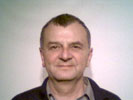• ## Basics of Convolution

See:Interactive Java Tutorial of the Florida States University
The filters presented in the previous chapters of this textbook can be divided into 3 types.

1. Linear filters: Simple Average, Gaussian
2. Nonlinear filters: Fast Average, Fast Gaussian, Median, 2x1 Laplace
3. Basically linear filters with nonlinear post processing: Sigma, Fog, 3x3 Laplace, Sobel

Type 1 filters and the linear part of the type 3 filters base on a common filter algorithm called discrete 2D spatial convolution or shortly convolution.
Convolution is a powerful instrument to improve images in many ways:

1. reducing random noise
2. sharpening the edges of image objects
3. correcting for unequal illumination
4. correcting for blur and motion artifacts

Such different imaging tasks can be carried out by one convolution algorithm which always follows these 3 steps:

1. Multiply the original image with an appropriate filter kernel.
2. Sum up the products.
3. Shift or divide and round the sum in order to receive output values in the range between `0` and `255`.

A problem with image convolution is the enormous number of multiplications and additions that need to be performed, often resulting in unacceptably long execution times.
Solution: Modern graphics cards contain special hardware processors that boost convolution.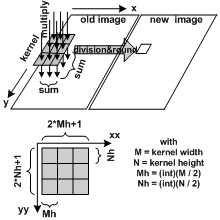Convolution unifies all sorts linear filtering: lowpass, highpass, bandpass. Basic algorithm: Define a small (not necessarily quadratic) matrix (called kernel) with uneven no. of rows and columns. Slide the kernel above the original image by adjusting its center in front of any pixel. At any place multiply any kernel value with its corresponding pixel and sum up the products. Finally shift, divide, round the sum in order to obtain an output pixel value. Advantages: All linear filters run the same algorithm. Specialized convolution-ASICs boost any linear filter. Disadvantages: A lot of redundant multiplications occur whenever the kernel values are `0` or `1`. Convolution is undefined at any image border where the kernel overlaps the border -> border problem.

The algorithm contains 4 `for` loops:

1. The 2 outer `for` loops iterate over the 2D input image `img0`.
2. The 2 inner `for` loops iterate over the small 2D kernel matrix.
3. The center of the kernel moves over any pixel of `img0`.
4. After any move all kernel values are multiplied with their underlying image pixels.
5. The products sum up and the sum is finally divided by a predefined `divisor >= 1`.
6. The result must by type converted to `Byte` and written into the output image `img1`.
7. The outer `mh` columns and `nh` rows of `img1` remain undefined.

Here is the pseudocode of convolution:

```const int m;  //no of kernel columns must be uneven >= 3
const int m;  //no of kernel rows must be uneven >= 3
const int mh = (m-1)/2, nh = (n-1)/2; //half wings lengths
float sum; const float divisor = ?.???; //divisor = sum of all kernel values (mostly)
for ( int y=nh; y < img0.Height-nh; y++ )
for ( int x=mh; x < img0.Width-mh; x++ )
{ sum = 0.0f;
for ( int yy=-nh; yy <= nh; yy++ )
for ( int xx=-mh; xx <= mh; xx++ )
sum += img0[y+yy,x+xx] * kernel[yy+nh,xx+mh];
img1[y,x] = Convert.ToByte( sum / divisor );
}```

# Experiments Try out the following kernels:

1. Average 3x3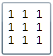Result: blurring
2. Edit the middle weight to 10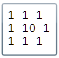Result: nearly no blurring
3. Edit the middle weight to -10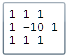Result: Sharpening highpass.Explanation: Flat areas remain untouched whereas any difference between center and periphery doubles.
4. Edit the middle weight to 8 and all others to -1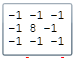Result: Edge detection highpass.Explanation: Nearly everywhere the colors sum up to 0.Colors just survive at sharp edges.
5. Edit all weights to 0.1Result: Same as Average 3x3.Explanation: Inside a kernel only relative differences count.
• ## C#-Code of Convolution

```WriteableBitmap img0, imgOut; //input and output images with 32-bit-ARGB-pixels
int xSize, ySize; //image width and height
float[,] kernel[n,m];  // m and n are uneven numbers e.g. 3, 5, 7, 9, 11, ...
//R0, G0, B0: intermediary 2D-arrays each containing a color plane of img0
Byte[,] R0[ySize,xSize], G0[ySize,xSize], B0[ySize,xSize];

private void Convolution()
{ int m = kernel.GetLength(0); //no of kernel columns
int n = kernel.GetLength(1); //no of kernel rows
int mh = (m-1) / 2; //horizontal half wing length
int nh = (n-1) / 2; //vertical   half wing length
int i, x, y, xx, yy, xxx, yyy, R, G, B;
float weight, sumR, sumG, sumB, divisor=0;
//Add all kernel values to obtain a divisor
for ( yy=0; yy < n; yy++ )
for ( xx=0; xx < m; xx++ ) divisor += kernel[yy,xx];
if ( divisor < 1f ) divisor = 1f; //divisor must be >= 1
//Preset all 32-bit-ARGB-pixels with A = ff and RGB = 000000
for ( i=0; i < xSize*ySize; i++ ) unchecked { imgOut.Pixels[i] = (Int32)0xff000000; }
//Here begins the convolution
for ( y=nh; y < ySize-nh; y++ )
{ i = y*xSize + mh; //linear index of the first pixel in row y
for ( x=mh; x < xSize-mh; x++, i++ ) //increment x and i
{ sumR = sumG = sumB = 0;
for ( yy=0; yy < n; yy++ )
{ yyy = y+yy-nh;
for ( xx=0; xx < m; xx++ )
{ xxx = x+xx-mh;
weight = kernel[yy,xx];
sumR += R0[yyy,xxx] * weight;
sumG += G0[yyy,xxx] * weight;
sumB += B0[yyy,xxx] * weight;
}
}
//Divide, convert to positive integer and clip to maximal 255
R = Math.Min(255, Convert.ToInt32(Math.Abs(sumR/divisor)));
G = Math.Min(255, Convert.ToInt32(Math.Abs(sumG/divisor)));
B = Math.Min(255, Convert.ToInt32(Math.Abs(sumB/divisor)));
//Shift the color bytes to their 32-bit-ARGB positions
imgOut.Pixels[i] += R << 16 | G << 8 | B;
}
}
}```

******************************************************************************
Q: What is linear Filter ? What is the basic difference to non-linear Filters ? Samples for both ?
A: A linear filter performs a convolution. Non-linear filters don't.
Linear samples: Average, Fog, Gaussian
Non-linear sample: Median
******************************************************************************
Q: What is a convolution ? Short description of the algorithm ?
A: Convolution is a mathematical operation on an image and a kernel, producing a modified version of the original image.

1. A small kernel matrix slides above the original image by adjusting its center in front of any pixel.
2. At any place multiply any kernel value with its corresponding pixel and sum up the products.
3. Finaly shift, divide, round the sum in order to obtain an output pixel value.

******************************************************************************
Q: What is a kernel ? Kernels of 3x3 Average-, Fog-, horizontal Laplace-filters ?
A: A matrix of figures with an uneven no of columns m >= 3 and an uneven no of rows n >= 3 used for convolution.

```          1 1 1        1  1 1                   -1 0 1
Average = 1 1 1; Fog = 1 -5 1; Horiz. Laplace = -2 0 2
1 1 1        1  1 1                   -1 0 1```

******************************************************************************
Q: Pseudocode of the convolution `img0 -> img1` ?

```A:
for ( all lines y of img0 )
for ( all columns x of img0 )
{ sum = 0.0f;
for ( all lines yy of the kernel )
for ( all columns xx of the kernel )
sum += img0[y+yy,x+xx] * kernel[yy,xx];
img1[y,x] = ConvertToColor( sum / divisor );
}```

******************************************************************************